• Call Now

1800-102-2727•

# Quadrilateral: Definition, Types, Parallelogram, Rhombus, Rectangle and Square

A quadrilateral is a topic in Euclidean geometry, which deals with polygons that contain four vertices and four sides. Quadrilaterals are of 2-dimensional structures. They can be classified into five major types. Trapezium The trapezium is a 2-dimensional shape that has a pair of parallel sides. NCERT Class 9 Maths Chapter 8 Quadrilaterals deals with the Angle Sum Property of a Quadrilateral, Types of Quadrilaterals such as Trapezium, Parallelograms, Rectangle, Rhombus, Square, and Kite. It further elucidates the Properties of a Parallelogram and Conditions for a Quadrilateral to be a Parallelogram.For example, in an isosceles trapezium, the angles on the opposite sides parallel to each other are equal. These angles are commonly known as the base angles.

Features of Trapezium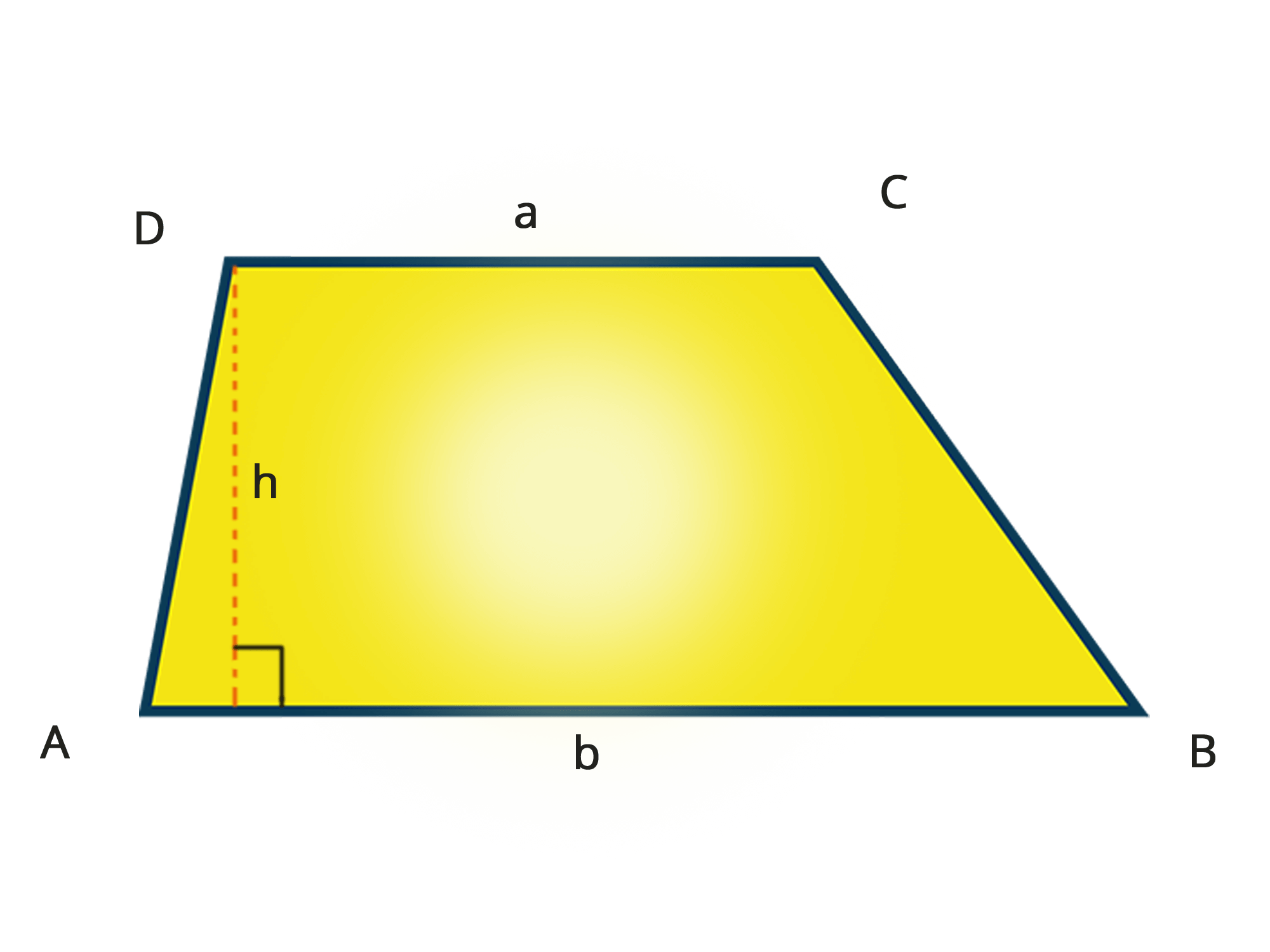• The trapezium’s base and the side opposite to it are parallel.
• The angles, diagonals and sides are not equal (except in the case of an isosceles trapezium).

## Parallelogram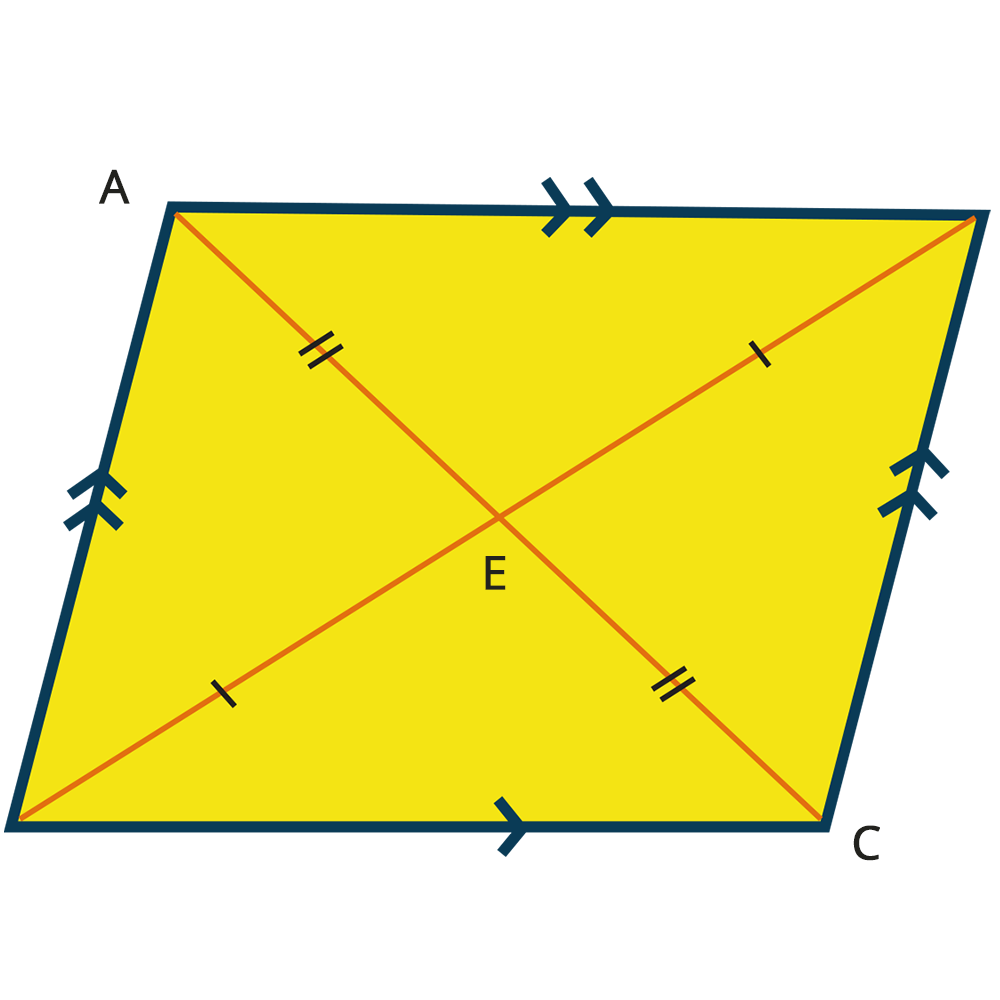A figure that has opposite sides parallel to each other is known as a parallelogram. Apart from that, the sides opposite to each other are equal.

Features of Parallelogram

• The opposite sides are equal as well as parallel to each other.
• They have congruent angles.
• The angles present in the adjacent side of theThe following are some of the important formulae for trapezium,
• Perimeter of Trapezium = L1+L2+L3+L4
• Area of Trapezium = 12 h (L1+L2)
• parallelogram are supplementary.
• If an angle present in the parallelogram is 90°, then by opposite angle property and the supplementary nature, other angles in the parallelogram are also 90°, making the parallelogram a rectangle.
• The diagonals of a parallelogram bisect each other and divide the figure into two congruent triangles.

Figure of a Parallelogram

The given below are some of the fundamental formulae related to a parallelogram
Perimeter of parallelogram = 2 (L + B)
Area of Parallelogram = L * H

## Rhombus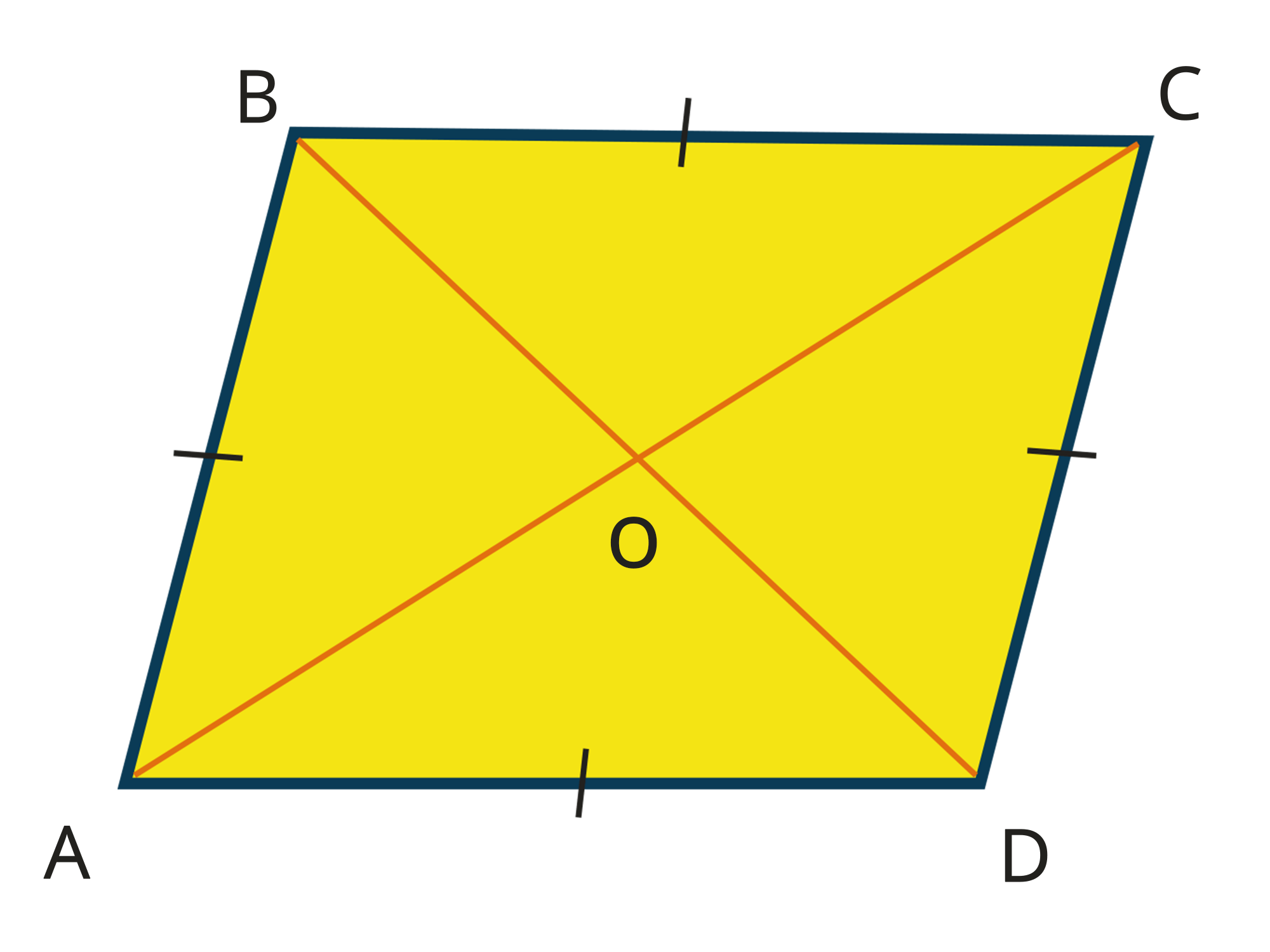A rhombus has all its four sides equal in terms of length. Therefore, diagonals present in a rhombus are perpendicular bisectors to one another.

Features of Rhombus

• The rhombus has all four equal sides.
• Rhombus has congruent angles on its opposite sides.
• The adjacent angles present are supplementary.
• Rhombus’s diagonals are perpendicular bisectors of each other.
• A rhombus is considered a special type of parallelogram.

If m and n are the diagonals of a rhombus, then,

Perimeter of Rhombus = 4 L
Area of Rhombus = (a * b) / 2

## Rectangle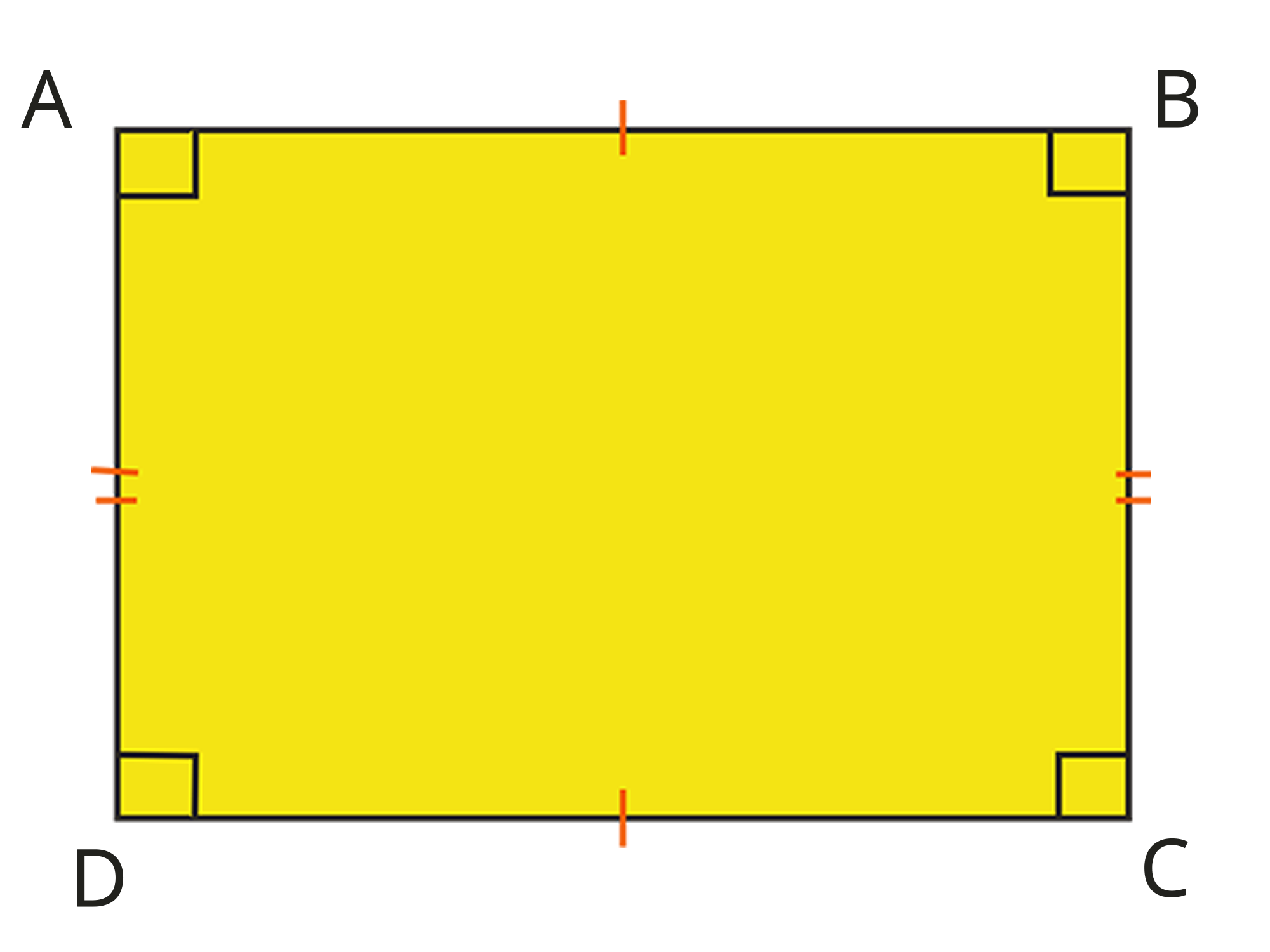A rectangle is another type of parallelogram that has all the angles equal to right angles. In a rectangle, opposite sides have the same length.

Properties of Rectangle

• Rectangle has its parallel opposite sides and is congruent.
• All angles contained in a rectangle are right angles.
• They have diagonals that bisect each other.

Following are the formulae related to rectangle,

Perimeter of Rectangle = 2 (L + B)
Area of Rectangle = L * B

## Square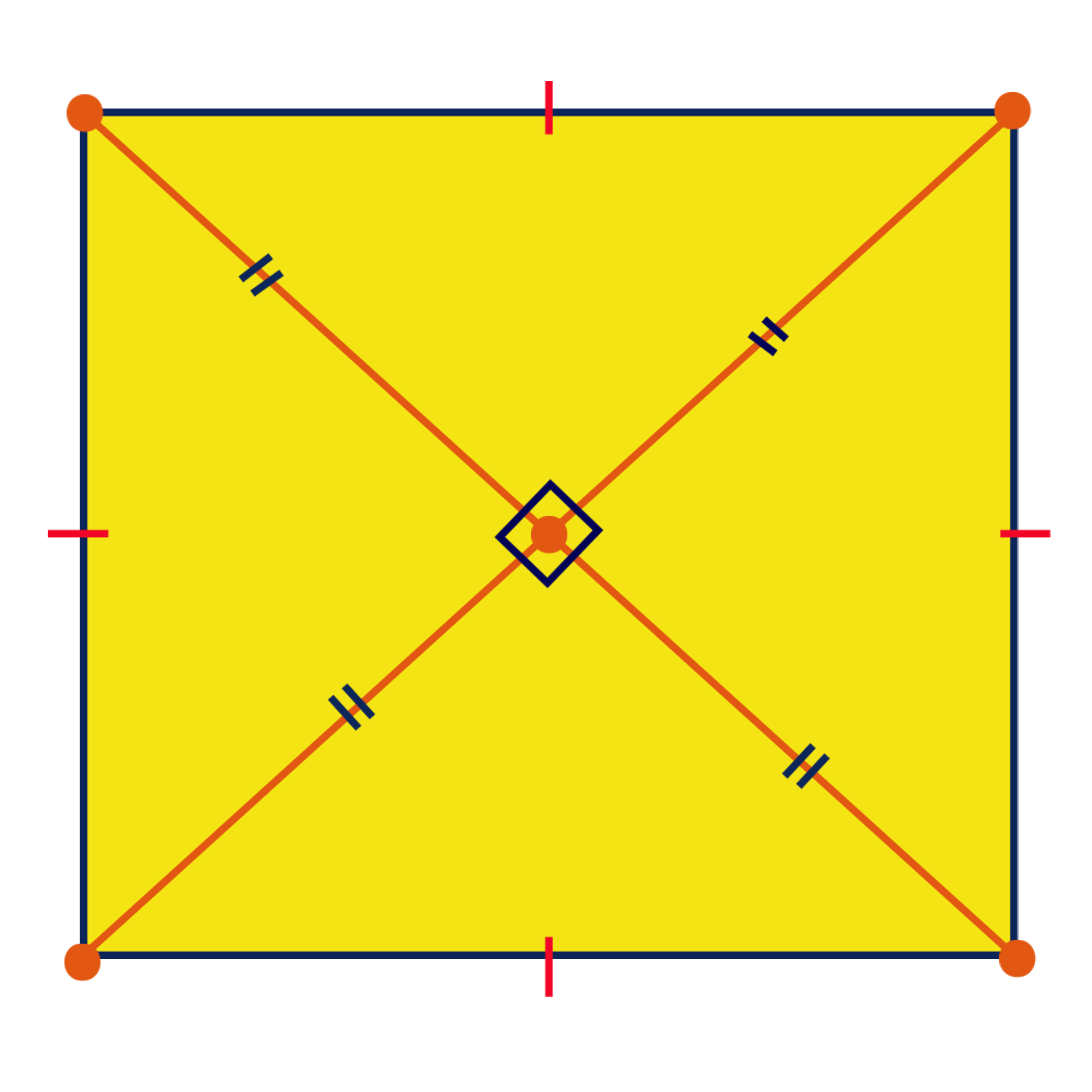A square is one of the most basic shapes in a quadrilateral that contains four equal sides in terms of length. All the four angles present in a square are also equal. Therefore, they are all right angles.
Properties of Square

• All angles and sides are congruent to one another.
• A square has parallel opposite sides.
• Diagonals present in a square are congruent as well.
• Diagonals are perpendicular bisectors of each other.
• A square can be regarded as a unique parallelogram with its angles and sides equal to each other.

Formulae related to the square are mentioned below,

Perimeter of Square = 4 L
Are of Square = L2Talk to our expert
Resend OTP Timer =
By submitting up, I agree to receive all the Whatsapp communication on my registered number and Aakash terms and conditions and privacy policy